Courses

# Notes | EduRev

## JEE : Notes | EduRev

The document Notes | EduRev is a part of the JEE Course JEE Revision Notes.
All you need of JEE at this link: JEE
• Work:-  Work done W is defined as the dot product of force F and displacement s.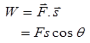Here θ is the angle between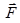and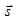.
Work done by the force is positive if the angle between force and displacement is acute (0°<θ<90°) as cos θ is positive. This signifies, when the force and displacement are in same direction, work done is positive. This work is said to be done upon the body.
• When the force acts in a direction at right angle to the direction of displacement (cos90° = 0), no work is done (zero work).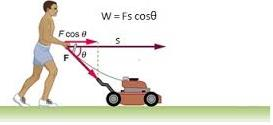• Work done by the force is negative if the angle between force and displacement is obtuse (90°<θ<180°)  as cosθ is negative. This signifies, when the force and displacement are in opposite direction, work done is negative. This work is said to be done by the body.
• Work done by a variable force:-
If applied force F is not a constant force, then work done by this force in moving the body from position A to B will be,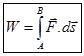Here ds is the small displacement.
• Units:  The unit of work done in S.I is joule (J) and in C.G.S system is erg.
1J = 1 N.m , 1 erg = 1 dyn.cm
• Relation between Joule and erg:- 1 J = 107 erg
• Power:-The rate at which work is done is called power and is defined as,
P = W/t = F.s/v = F.v
Here s is the distance and v is the speed.
• Instantaneous power in terms of mechanical energy:- P = dE/dt
• Units: The unit of power in S.I system is J/s (watt) and in C.G.S system is erg/s.
• Energy:-
1) Energy is the ability of the body to do some work. The unit of energy is same as that of work.
2) Kinetic Energy (K):- It is defined as,
K= ½ mv2
Here m is the mass of the body and v is the speed of the body.
• Potential Energy (U):- Potential energy of a body is defined as, U = mgh
Here, m is the mass of the body, g is the free fall acceleration (acceleration due to gravity) and h is the height.
• Gravitational Potential Energy:- An object’s gravitational potential energy U is its mass m times the acceleration due to gravity g times its height h above a zero level.
In symbol’s,
U = mgh
• Relation between Kinetic Energy (K) and momentum  (p):-
K = p2/2m
• If two bodies of different masses have same momentum, body with a greater mass shall have lesser kinetic energy.
• If two bodies of different mass have same kinetic energy, body with a greater mass shall have greater momentum.
• For two bodies having same mass, the body having greater momentum shall have greater kinetic energy.
• Work energy Theorem:- It states that work done on the body or by the body is equal to the net change in its kinetic energy .
• For constant force,
W = ½ mv– ½ mu2
= Final K.E – Initial K.E
• For variable force,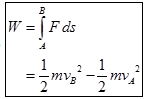• Law of conservation of energy:- It states that, “Energy can neither be created nor destroyed. It can be converted from one form to another. The sum of total energy, in this universe, is always same”.
• The sum of the kinetic and potential energies of an object is called mechanical energy. So, E = K+U
• In accordance to law of conservation of energy, the total mechanical energy of the system always remains constant.
So, mgh + ½ mv2 = constant
In an isolated system, the total energy Etotal of the system is constant.
So, E = U+K = constant
Or, Ui+Ki = Uf+Kf
Or, ?U = -?K
Speed of particle v in a central force field:
v = √2/m [E-U(x)]
• Conservation of linear momentum:-
In an isolated system (no external force ( Fext = 0)), the total momentum of the system before collision would be equal to total momentum of the system after collision.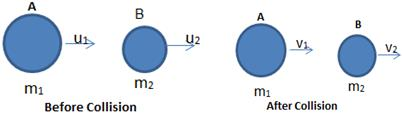So, p= pi
• Coefficient of restitution (e):- It is defined as the ratio between magnitude of impulse during period of restitution to that during period of deformation.
e = relative velocity after collision / relative velocity before collision
= v– v1/u1 – u2
Case (i) For perfectly elastic collision, e = 1. Thus, v2 – v1 = u– u2. This signifies the relative velocities of two bodies before and after collision are same.
Case (ii) For inelastic collision, e<1. Thus, v2 – v1 < u1 – u2. This signifies, the value of e shall depend upon the extent of loss of kinetic energy during collision.
Case (iii) For perfectly inelastic collision, e = 0. Thus, v2 – v=0, or v2 = v1. This signifies the two bodies shall move together with same velocity. Therefore, there shall be no separation between them.
• Elastic collision:- In an elastic collision, both the momentum and kinetic energy conserved.
• One dimensional elastic collision:-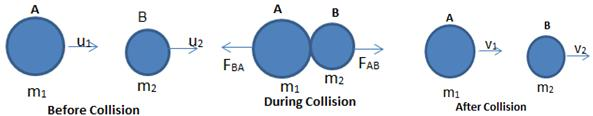After collision, the velocity of two body will be,
v1 = (m1-m2/ m1+m2)u1 + (2m2/ m1+m2)u2
and
v2 = (m2-m1/ m1+m2)u+ (2m1/ m1+m2)u1
Case:I
When both the colliding bodies are of the same mass, i.e., m1 = m2, then,
v1 = u2 and v2 = u1
Case:II
When the body B of mass m2 is initially at rest, i.e., u2 = 0, then,
v1 = (m1-m2/ m1+m2)u1 and v= (2m1/ m1+m2)u1
(a) When  m2<<m1, then, v1 = u1 and v2 = 2u1
(b) When  m2=m1, then, v1 =0  and v2 = u1
(c) When  m2>>m1, then, v= -u1 and v2 will be very small.
• Inelastic collision:- In an inelastic collision, only the quantity momentum is conserved but not kinetic energy.
v = (m1u1+m2u2) /(m1+m2)
and
loss in kinetic energy, E = ½ m1u12+ ½ m2u22 - ½ (m1+ m2)v2
or,
E= ½ (m1u12 + m2u22) – ½ [(m1u1+ m2u2)/( m1+ m2)]2
= m1 m2 (u1-u2)/ 2( m1 + m2)
• Points to be Notice:-
(i) The maximum transfer energy occurs if m1= m2
(ii) If Kis the initial kinetic energy and Kf is the final kinetic energy of mass m1, the fractional decrease in kinetic energy is given by,
K– Kf / Ki = 1- v12/u21
Further, if m= nm1 and u2 = 0, then,
Ki – Kf / K= 4n/(1+n)2
• Conservation Equation:
(i)  Momentum – m1u1+m2u2 = m1v1+m2v2
(ii) Energy – ½ m1u12+ ½ m2u22 = ½ m1v12+ ½ m2v22
• Conservative force (F):- Conservative force is equal to the negative gradient of potential V of the field of that force. This force is also called central force.
So,  F = - (dV/dr)
• The line integral of a conservative force around a closed path is always zero.
So,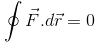• Spring potential energy (Es):- It is defined as,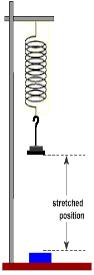E= ½ kx2
Here k is the spring constant and x is the elongation.
• Equilibrium Conditions:
(a) Condition for equilibrium, dU/dx = 0
(b) For stable equilibrium,
U(x) = minimum,
dU/dx = 0,
d2U/dx2 = +ve
(c) For unstable equilibrium,
U(x) = maximum
dU/dx = 0
d2U/dx2 = -ve
(d) For neutral equilibrium,
U(x) = constant
dU/dx = 0
d2U/dx= 0

• UNITS AND DIMENSIONS OF WORK, POWER AND ENERGY
Work and Energy are measured in the same units. Power, being the rate at which work is done, is measured in a different unit.
 Quantity and  Units/Dimensions Work (Energy) Power Dimension ML2T-2 ML2T-3 Absolute MKS Joule Watt FPS ft-Poundal ft-poundal/sec CGS erg Erg/sec. Gravitational MKS kg-m Kg-m/sec FPS ft-lb ft-lb/sec. CGS gm-cm Gm-cm/sec Practical(Other) kwh, eV, cal HP, kW, MW
• Conversions between Different Systems of Units
1 Joule = 1 Newton ´ 1 m = 105 dyne ´ 102 cm = 107 erg
1 watt = 1 Joule/ sec = 107 erg/sec.
1 kwh  = 103 watt ´ 1 hr  = 103 watt ´ 3600 sec
= 3.6 ´ 106 Joule
1HP = 746 watt.
1 MW = 106 watt.
1 cal = 1 calorie = 4.2 Joule
1eV = "e" Joule  = 1.6 ´ 10-19 Joule
(e = magnitude of charge on the electron in coulombs)
Offer running on EduRev: Apply code STAYHOME200 to get INR 200 off on our premium plan EduRev Infinity!

233 docs

,

,

,

,

,

,

,

,

,

,

,

,

,

,

,

,

,

,

,

,

,

;